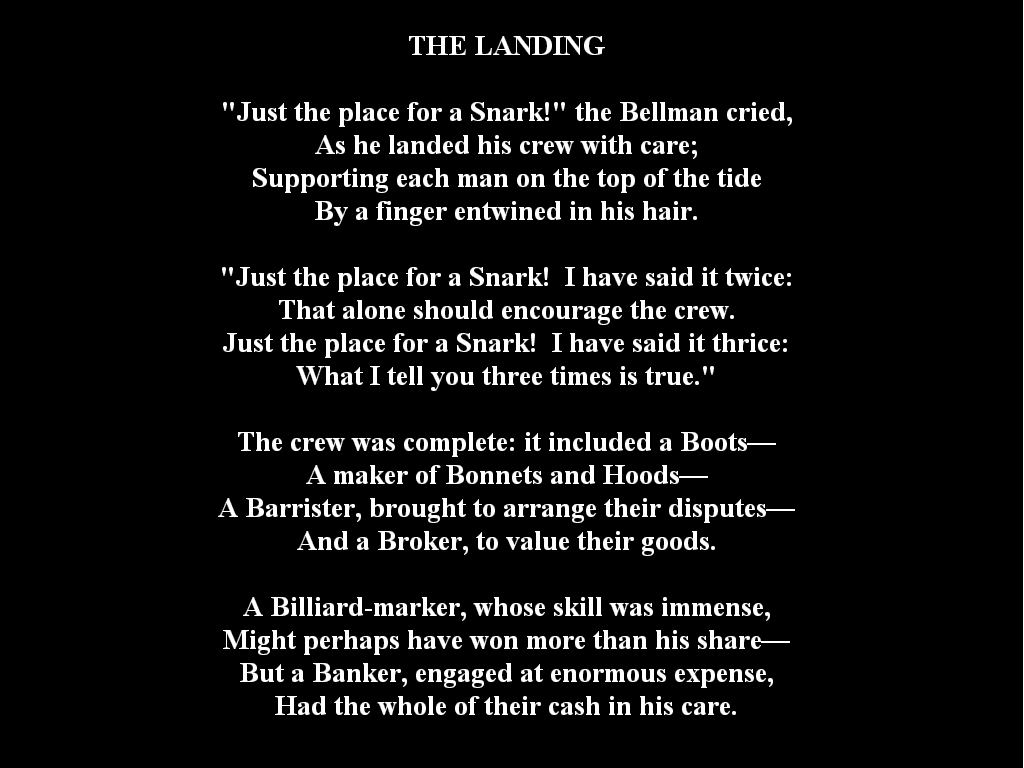# BioEye 2015

## Competition on Biometrics via Eye Movements

#### An official competition of BTAS 2015New user? Register

### Information for the Database

You can download the development datasets from here (Currently unavailable).
You can download the evaluation datasets from here (Currently unavailable).

#### Database Formation

##### Recordings
The database recordings were implemented in three sessions. The first and the second session were performed with the participation of a total of 306 subjects (ages 18-43). The first two sessions were separated by a time interval of 30 minutes. A third recording session was performed from a subset of 74 subjects from the initial subject pool. The time interval between the third recording session and the two first sessions was 1 year. The device used to implement the recordings was an EyeLink eye-tracker working at 1000 Hz, with a vendor-reported spatial accuracy of 0.5 degrees. During the recordings, only the left eye was captured.
##### Stimuli
Two different visual stimuli were used for the recordings: a) Random dot stimulus (RAN). In this case, the stimulus consisted of a white dot appearing at random positions in a black background in a computer screen. The white dot changed position every 1 second, and the total recording time was 1 minute and 40 seconds. An example of the used random dot stimulus is shown in Figure 1. b) Text stimulus (TEX). In this case, the participants read freely some text excerpts from the poem of Lewis Carroll “The Hunting of the Snark”. The total time that was given to the participants to read the excerpts was 1 minute. An example of the used text stimulus is shown in Figure 2.

Visual stimuli were presented on a flat screen monitor positioned at a distance of 550 mm from each subject, with dimensions of 474×297 mm, and screen resolution of 1680×1050 pixels.Figure 1: Example of the used random dot stimulus (RAN).Figure 2: Example of the used text stimulus (TEX).
##### Data Quality

The recordings were implemented following a rigorous protocol for ensuring maximum data quality. Also, the subjects’ heads were stabilized with the use of a chin-rest.

The average calibration accuracy (CAL) values calculated after the completion of recordings were: for the case of RAN_30min, CAL = 0.49 deg. (STD = 0.17 deg.), for the case of RAN_1year, CAL = 0.48 deg. (STD = 0.15 deg.), for the case of TEX_30min, CAL = 0.48 deg. (STD = 0.17 deg.), for the case of TEX_1year, CAL = 0.47 deg. (STD = 0.16 deg.).

The average recorded data validity (VAL) values calculated after the completion of recordings were: for the case of RAN_30min, VAL = 96.59% (STD = 4.86%), for the case of RAN_1year, VAL = 97.18% (STD = 3.47%), for the case of TEX_30min, VAL = 94.26% (STD = 5.76%), for the case of TEX_1year, VAL = 93.84% (STD = 5.82%).

##### Pre-processing

The raw eye movement signals were subsampled at a frequency of 250 Hz (anti-aliasing filter was used). Signal subsampling at this frequency was considered suitable for the following reasons: i) the eye movement signal characteristics are not distorted (verified though experimental analysis), ii) the resulting size of each recording (total number of samples) can be easily processed by signal processing algorithms, iii) the required storage space for the complete database is kept at a reasonable size.

#### Database Structure

##### Included Datasets
The recordings were organized in datasets according to the used visual stimuli and the time interval between the recordings. This led to the formation of the four datasets, described below:

1. RAN_30min: This dataset contains 2 recordings per subject, recorded with a 30 minute time interval, and by using the random dot stimulus (RAN).
2. TEX_30min: This dataset contains 2 recordings per subject, recorded with a 30 minute time interval, and by using the text stimulus (TEX).
3. RAN_1year: This dataset contains 2 recordings per subject, recorded with an 1 year time interval, and by using the random dot stimulus (RAN).
4. TEX_1year: This dataset contains 2 recordings per subject, recorded with an 1 year time interval, and by using the text stimulus (TEX).

Each dataset was split in two groups, one for the development phase and one for the evaluation phase of the competition, designated with the suffixes dv and ev (see also Procedure page).

##### Files Structure
The name of each file is in the form: ID_SubjectNum_SessionNum.txt. Here, SubjectNum is the ID number of the subject and SessionNum is the corresponding recording session, for example ID_001_1.txt, ID_053_2.txt, ID_201_3.txt etc.

The .txt files are written in plain ASCII format. The first line of the file is a header with the description of the fields. The other lines contain the samples. All samples consist of 6 fields:

1. SAMPLE: Sequential eye position number.
2. X DEGREE: Horizontal eye position in degrees of visual angle. Center of the screen corresponds to position (0,0).
3. Y DEGREE: Vertical eye position in degrees of visual angle.
4. VALIDITY: Data validity flag (1 for valid data and 0 for invalid data).
5. X STIMULI: Horizontal stimuli position in degrees of visual angle. *
6. Y STIMULI: Vertical stimuli position in degrees of visual angle. *

That makes easy to read the content of the file. For example, in MATLAB it can be done by executing:
FID = fopen( filename, 'rt');
if( FID ~= -1 )
TextData = textscan( FID, '%d %f %f %d %f %f', 'HeaderLines', 1);
fclose(FID);
end;

Here, filename is the full filename for the input file, and TextData is a cell array that contains the data from the file.

* Note that the values for X STIMULI and Y STIMULI fields are provided only for the Random dot stimulus (RAN), since the Text reading stimulus (TEX) was a free viewing task. If you are interested in the exact content and layout of the text stimulus (TEX), you can download the corresponding .png files from here (you must be logged in).